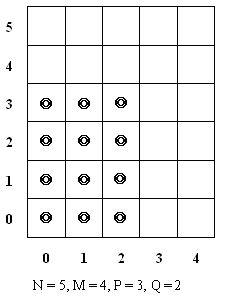Online JudgeProblem SetAuthorsOnline ContestsUser
Web Board
F.A.Qs
Statistical Charts
Problems
Submit Problem
Online Status
Prob.ID:
Register
Authors ranklist
Current Contest
Past Contests
Scheduled Contests
Award Contest
Register
Language:
A New Kind of Chess
 Time Limit: 3000MS Memory Limit: 65536K Total Submissions: 370 Accepted: 57

Description

Prince Remmarguts met Uyuw successfully in our previous story, and after that Princess Uyuw introduced a new kind of chess to Remmarguts named Nixgnauhc. The only chessman allowed in it was a special type of Knight.

The chessboard is of (N + 1) * (M + 1). Each of the rows and columns are numbered as the following graph:Here N + 1 = 5 + 1 is the number of rows and M + 1 = 4 + 1 is the number of columns. We are also given two integer numbers P and Q, and told that at the beginning of the game, the blocks of (x, y) - (row number, column number) - where x <= P and y <= Q are already taken up by Knight.
During the game, we can choose a single Knight to move, and the only allowed movements for Knight at (x, y) is to (x + a, y + b) or to (x + c, y + d). But during the movement, the position it goes must be on the chessboard and NOT be taken up by another Knight. Our purpose is to move that chessman to the final end (N, M). (The description above means that once you choose a chessman, you can only move that chessman in the following steps)

Meanwhile, we suppose 3 <= N, M <= 100000, 0 <= P < N, 0 <= Q < M, 1<= a, b, c, d. Princess Uyuw wanted to know the number of essentially different games. Two games are called “different” if and only if we choose the different chessman at the beginning or perform a different movement at some time.

WARNING: Even if a = c, b = d, we also call (+a, +b) and (+c, +d) DIFFERENT movements!

Input

You should read the number of test cases Z in the first line – Z <= 100.

Each of the following lines denotes a single test case, consisting of 8 integers N, M, P, Q, a, b, c, and d. The meanings of such integers are described above.

Output

Output one line per test case, showing the total possibilities of games. We guarantee this number is less than 10^500.

Sample Input

```2
3 3 0 0 1 1 1 1
5 4 2 1 1 1 2 1
```

Sample Output

```8
7
```

Source

POJ Monthly,Zeyuan Zhu

[Submit]   [Go Back]   [Status]   [Discuss]Home PageGo BackTo top